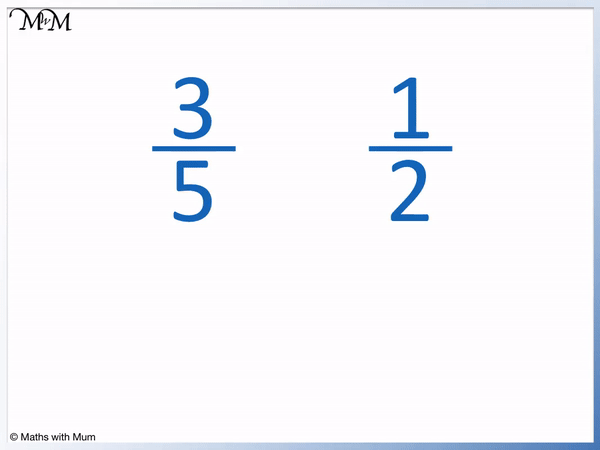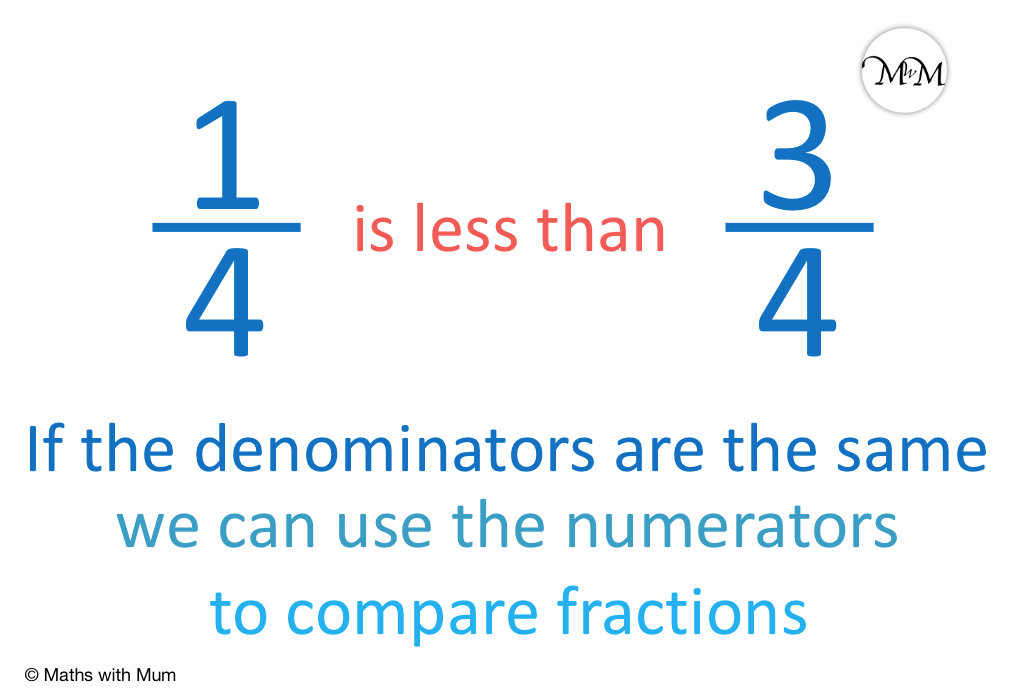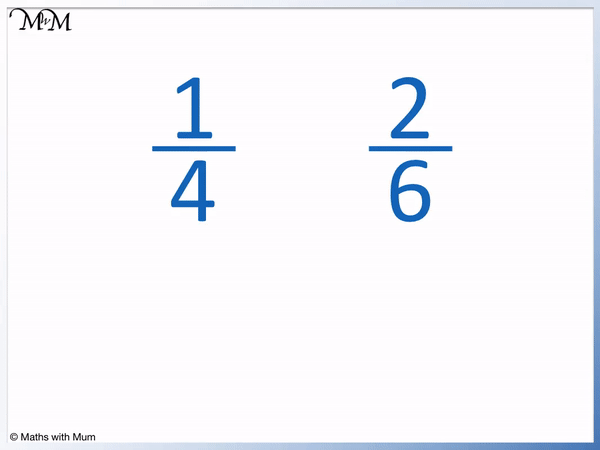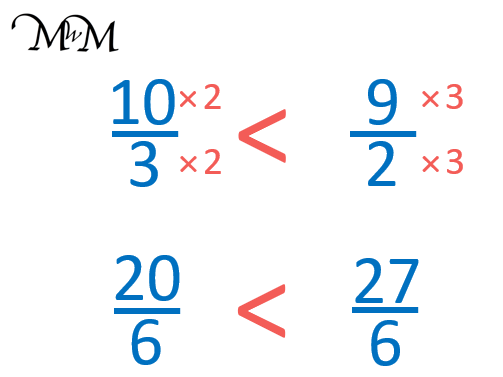# How to Compare Unlike Fractions

How to Compare Unlike Fractions• To compare two fractions, find equivalent fractions with a common denominator. Then order the fractions by their new numerators on top.
• 3 / 5   and 1 / 2   have denominators on the bottom of 5 and 2 respectively.
• A common denominator is chosen which is the first number in the 5 and 2 times table. This number is 10.
• We multiply the numerator on top and the denominator on the bottom by the same number to make equivalent fractions which have common denominators.
• Multiplying top and bottom by 2,   3 / 5   =   6 / 10  .
• Multiplying top and bottom by 5,   1 / 2   =   5 / 10  .
• Both fractions are now out of 10, so we can compare them.
• 6 / 10   is greater than   5 / 10   because 6 is greater than 5.
• Therefore,   3 / 5   is greater than   1 / 2  .
• We write this as   3 / 5   >   1 / 2  .
• The inequality sign of ‘>’ always points at the smaller number

Find equivalent fractions with a common denominator.

Then the fraction with the largest numerator on top is the biggest.• 2 / 3   and   3 / 5   have denominators of 3 and 5 respectively.
• The first number in both the 3 and 5 times table is 15 and so, the common denominator will be 15.
• We multiply the top and bottom by 5 so that   2 / 3   =   10 / 15   .
• We multiply the top and bottom by 3 so that   3 / 5   =   9 / 15   .
• Now that both fractions have the same denominator, we compare them by comparing the size of their numerators.
• 10 / 15   is greater than   9 / 15   because 10 is greater than 9.
• Therefore   2 / 3   is greater than   3 / 5  .
• We write this as   2 / 3   >   3 / 5  , with the sign pointing at the smaller fraction.#### How to Compare Fractions with Different Denominators# Comparing Fractions with Different Denominators

## What are Unlike Fractions?

Unlike fractions are two or more fractions that have different denominators. This means that the numbers on the bottom of unlike fractions are different. Unlike fractions are each divided into different-sized parts and so, they cannot be compared or added easily.

For example,   1 / 4   and   1 / 3   are unlike fractions because the denominators are different. The first fraction is divided into 3 parts and the second fraction is divided into 4 parts.

Like fractions are fractions that have the same denominator on the bottom. For example,   1 / 4   and   3 / 4  . These fractions both have the same denominator of 4.We can see that   3 / 4   is larger than   1 / 4   because we have 3 quarters compared to 1 quarter. We are comparing parts that are the same size.

However, it is not immediately clear which is larger out of the unlike fractions of   2 / 3   and   3 / 5  .

## How to Compare Fractions with Unlike Denominators

To compare fractions with different denominators, follow these steps:

1. Find the first number to appear in the times table of every denominator. This is the common denominator.
2. Write each fraction as an equivalent fraction that has this common denominator.
3. The larger the numerator of these fractions, the larger the fraction.

As an example, we will compare the unlike fractions of   1 / 4   and   2 / 6  .

Step 1 is to find the first number to appear in the times table of both denominators. The 4 times table is 4, 8, 12, 16, 20, 24. The 6 times table is 6, 12, 18, 24.

We only need to list the numbers up to 24 because 4 × 6 = 24. However We can see that 12 is a number that appears first. We will use 12 as the common denominator.Step 2 is to write both fractions as equivalent fractions with a common denominator. We will write both fractions out of 12.

1 / 4   =   3 / 12   and   2 / 6   =   4 / 12  .

Step 3 is to compare the fractions using their numerator.

3 is less than 4 and so,   4 / 12   is less than   3 / 12  .

This allows us to compare the original fractions.

1 / 4   is less than   2 / 6  .

We can write this as   1 / 4   <   2 / 6  .

We can use the inequality signs of greater less and less than to compare fractions. The sign of ‘<' or '>‘ always opens up to face the larger fraction and points at the smaller fraction.

Here is another example of comparing fractions with different denominators. We have   3 / 4   and   7 / 8  .We can see that 8 is the first number in the 4 and 8 times table. We double the numbers in the first fraction so that   3 / 4   =   6 / 8  .

We can keep   7 / 8   the same.

Now that both fractions are out of 8, we can compare them.

6 is less than 7 and so,   6 / 8   is less than   7 / 8  .

Therefore we can say that   3 / 4   is less than   7 / 8  .

We write   3 / 4   <   7 / 8  . The arrow of the sign points to the smaller fraction.

## How to Compare Improper Fractions with Different Denominators

To compare improper fractions with different denominators, first write each fraction as its equivalent fraction so that they both have the same denominator. The largest improper fraction will now have the largest numerator. Two improper fractions can only be compared if they have the same denominator on the bottom.

For example, here are the improper fractions of   5 / 4   and   6 / 5  . Improper fractions are simply fractions that have a larger numerator on top than their denominator on the bottom.The common denominator of these fractions is 20. 20 is the first number in both the 4 and 5 times table.

Writing the fractions as equivalent fractions with denominators of 20,   5 / 4   =   25 / 20   and   6 / 5   =   24 / 20  .

25 is a larger numerator than 24 and so,   25 / 20   is larger than   24 / 20  .

Therefore   5 / 4   is greater than   6 / 5  .

Here is another example of comparing the size of two improper fractions.

We have   10 / 3   and   9 / 2  .We write   10 / 3   =   20 / 6   and   9 / 2   =   27 / 6  .

Both fractions have the same denominator and can now be ordered.

20 is less than 27 and so,   20 / 6   is less than   27 / 6  .

Therefore   10 / 3   is less than   9 / 2  .

## Comparing Unlike Fractions using Decimals

Converting unlike fractions to decimals is a method that can be used to compare the size of them. Fractions can be turned into decimals by dividing the numerator by the denominator. The larger the decimal number, the larger the fraction.

For example, here we have the fractions   2 / 5   and   3 / 4  .

We turn   2 / 5   into a decimal by dividing 2 by 5.

2 ÷ 5 = 0.4.

We turn   3 / 4   into a decimal by dividing 3 by 4.

3 ÷ 4 = 0.75.The larger decimal number is 0.75. Therefore the larger fraction is   3 / 4  .

We say that   2 / 5   is less than   3 / 4Now try our lesson on Adding Fractions with Unlike Denominators where we learn how to add two fractions.